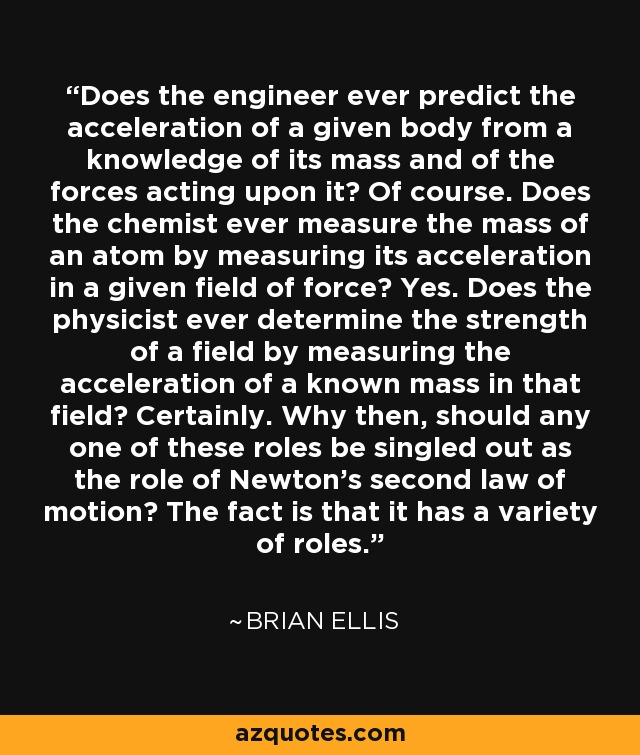Authors:
• Does the engineer ever predict the acceleration of a given body from a knowledge of its mass and of the forces acting upon it? Of course. Does the chemist ever measure the mass of an atom by measuring its acceleration in a given field of force? Yes. Does the physicist ever determine the strength of a field by measuring the acceleration of a known mass in that field? Certainly. Why then, should any one of these roles be singled out as the role of Newton's second law of motion? The fact is that it has a variety of roles.

### TopicsEmbed: